# Index of /laurent/DecimalsRangeRegexpGenerator

```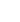Name                    Last modified      Size  Description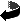Parent Directory                             -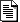app.html                2022-12-30 19:04  120K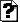README.md               2022-12-30 19:04  6.7KREADME.html             2022-12-30 19:10  8.0KLICENSE                 2022-11-27 23:52   34KCOPYING                 2022-11-27 23:52   34K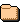.idea/                  2022-12-30 19:04    -.gitignore              2022-11-27 23:52    7.git/                   2022-12-30 19:04    -
```

This file is part of DecimalsRangeRegexpGenerator.

DecimalsRangeRegexpGenerator is free software: you can redistribute it and/or modify it under the terms of the GNU Affero General Public License as published by the Free Software Foundation, either version 3 of the License, or (at your option) any later version.

DecimalsRangeRegexpGenerator is distributed in the hope that it will be useful, but WITHOUT ANY WARRANTY; without even the implied warranty of MERCHANTABILITY or FITNESS FOR A PARTICULAR PURPOSE. See the GNU Affero General Public License for more details.

You should have received a copy of the GNU Affero General Public License along with DecimalsRangeRegexpGenerator. If not, see https://www.gnu.org/licenses/

# DecimalsRangeRegexpGenerator

Handle min, max and step to produce a regular expression for ranges of decimal numbers.

Several integers range regular expression generators can be found on GitHub, but they do not handle decimal numbers. (Decimal numbers are the subset of the rational numbers that can be written as n/10^k where n and k are integers.) They can handle min and max parameters, but they do not handle step parameter.

The goal of this dev tool is to have an easy path to avoid most use cases of HTML’s:

``<input type="number" min="@some_decimal1@" max="@some_decimal2@" step="@some_decimal3@">``

by using

``<input type="text" inputmode="numeric" pattern="@some_regexp@">``

For a few reasons to replace input number with input text and a pattern, you may read the following blog articles or webpages:

## Current features

So far, you can customize your regular expression with the following optional parameters:

• a min value that can be an arbitrary decimal number,
• a max value that can be an arbitrary decimal number,
• a step value that works currently:
• efficiently for numbers that can be written as 1/b^k, like 100 or 0.01,
• more or less efficiently for other steps: your mileage may vary but do not expect great results if your step has many significant digits,
• allow or not leading zeros,
• allow or not trailing zeros,
• a min (positive or zero integer) number of digits before decimal separator,
• a max (positive or zero integer) number of digits before decimal separator,
• a min (positive or zero integer) number of digits after decimal separator,
• a max (positive or zero integer) number of digits after decimal separator,
• apply or not min number of digits after decimal part if there is no decimal part,
• a min (positive or zero integer) number of digits before or after decimal separator,
• a max (positive or zero integer) number of digits before or after decimal separator,
• allow or not zero to be written with a minus sign,
• allow or not zero to be written with a plus sign,
• allow or not numbers above zero to be written with a plus sign.

Moreover, you can change the number of digits (your base) and the unicode characters you use for:

• digits,
• decimal separators,
• minus signs,
• plus signs.

With a number of digits different of ten, you are not dealing with decimal numbers anymore. You look at numbers that can be written as n/b^k where n and k are integers and b is your base (the number of digits you specified). Try the digits “0🐛” for “buginary” encoding ;).

Spacing is supported with:

• spaces characters allowed before a sign character
• a min (positive or zero integer) number of spaces before the sign,
• a max (positive or zero integer) number of spaces before the sign,
• spaces characters allowed before a number without sign,
• a min (positive or zero integer) number of spaces before the number,
• a max (positive or zero integer) number of spaces before the number,
• spaces characters allowed between a sign and a number (you may use non-breakable space here for example),
• a min (positive or zero integer) number of spaces between a sign and a number,
• a max (positive or zero integer) number of spaces between a sign and a number,
• spaces characters allowed after last_digit,
• a min (positive or zero integer) number of spaces after last_digit,
• a max (positive or zero integer) number of spaces after last_digit,
• spaces characters between digits before decimal separator,
• a min (positive or zero integer) number of spaces characters between digits before decimal separator,
• a max (positive or zero integer) number of spaces characters between digits before decimal separator,
• spaces characters between digit and decimal separator,
• a min (positive or zero integer) number of spaces characters between digit and decimal separator,
• a max (positive or zero integer) number of spaces characters between digit and decimal separator,
• spaces characters between decimal separator and digit,
• a min (positive or zero integer) number of spaces characters between decimal separator and digit,
• a max (positive or zero integer) number of spaces characters between decimal separator and digit,
• spaces characters between digits after decimal separator,
• a min (positive or zero integer) number of spaces characters between digits after decimal separator,
• a max (positive or zero integer) number of spaces characters between digits after decimal separator,
• a min (positive integer) number of contiguous digits,
• a max (positive integer) number of contiguous digits, you can set both to 3 for thousands separators.

There is also one parameter:

• a choice to enclose simple groups in regular expression with capturing or non-capturing groups.

app.html is hosted there on my website: https://lyaudet.eu/laurent/DecimalsRangeRegexpGenerator/app.html Since it is only static HTML and JS, it should handle many users :). Otherwise, you can just save the file on your computer, and open it in your web-browser to use it.

If you are happy with GNU AGPL, I welcome contributions to this project for the following things to be done:

• improve step regular expression generation with special cases and techniques to simplify it: for a number n/b^k, the regular expression should be at least linear in n, most of the time, which means exponential in the writing of n; hence, it comes with practical limits; but it would still be nice to be able to have a step like 0.25, for example, working with a small regular expression;
• add some testing code and enhance UI;
• other ideas you may have?

Please, email me, if you are interested.